• 以上网络图均由亿图图示软件绘制而成 接下来我直接演示绘制过程： 第一步，打开软件，选择“新建>网络图”，根据自己要绘制的图形类别进一步选择。 亿图图示提供了AWS、Azure、GCP、基本网络图、思科网络...
简单易用
绘图美观
兼容Windows和Mac
那么，亿图图示会是不错的选择！

贴图为证：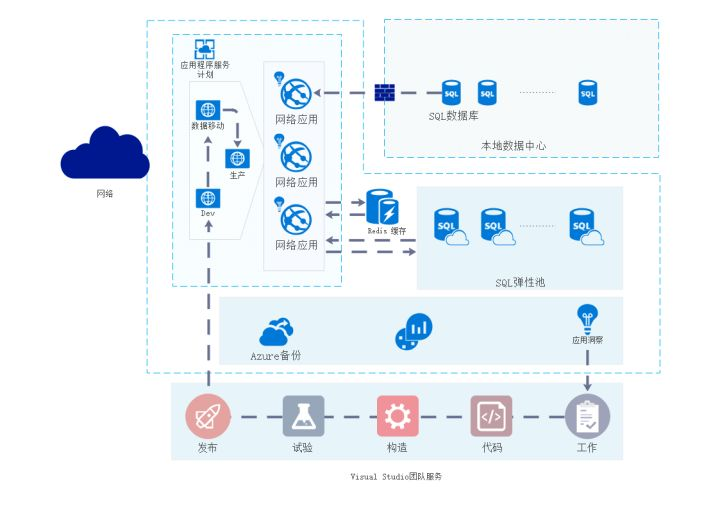Azure网络图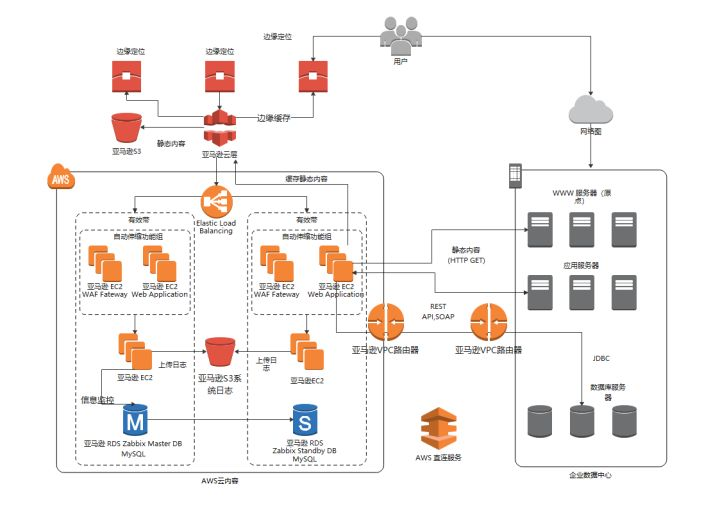AWS网络图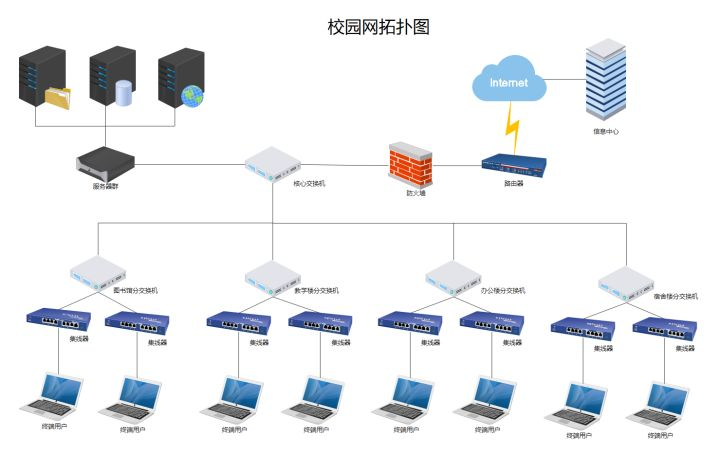校园网络拓扑图

以上网络图均由亿图图示软件绘制而成

接下来我直接演示绘制过程：

第一步，打开软件，选择“新建>网络图”，根据自己要绘制的图形类别进一步选择。

亿图图示提供了AWS、Azure、GCP、基本网络图、思科网络拓扑图等多种网络图图形；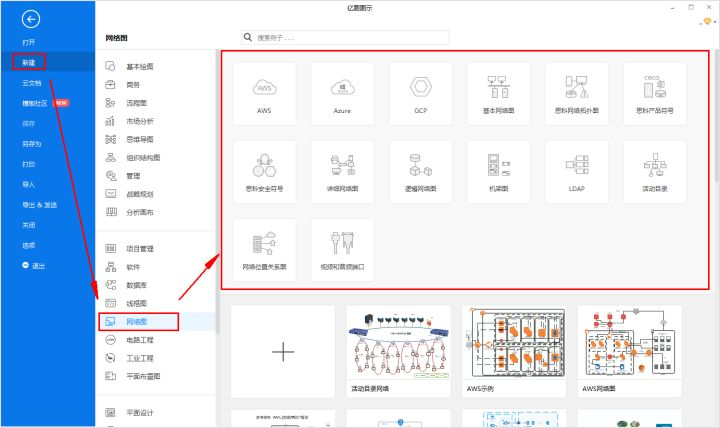新建画布

第二步，从左侧的符号库拖放图形到画布合适的位置，支持图形自由旋转、缩放、换色等操作；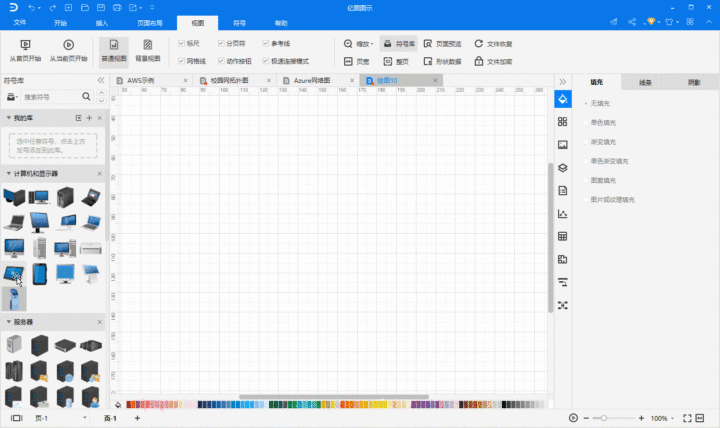符号自由拖放

亿图图示拥有丰富的图形符号库，26000+个矢量图形，大大省去了你绘制单个组件所需的时间。

比如网络图类型下的符号库，就分为计算机和显示器、服务器、网络和外设、详细网络图、安控设备、活动目录等类型，每种类型下都拥有众多图形符号，基本能满足你的绘图需求。

值得一提的是，最新版亿图图示还增加了“自定义符号库”的新功能，支持导入图片、SVG、EDT符号库等文件，满足用户更多样的绘图需求。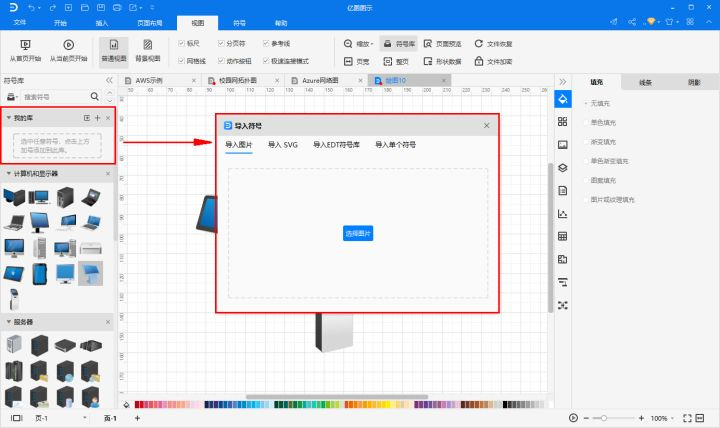自定义符号库

第三步，选择合适的连接线，表达出该网络系统中各种设备的关系。连线

简单三步，一幅网络图就完成了。

感兴趣的小伙伴，可以自己体验下

当然，如果你想绘图更快，可以考虑亿图图示的模板中心，选择合适的网络图模板，支持一键使用，非常方便

展开全文• ## Python 利用NetworkX绘制精美网络图

万次阅读 多人点赞 2020-08-08 10:30:31
Python数据分析，NetworkX 是一个用Python语言开发的图论与复杂网络建模工具，内置了常用的图与...本文简要介绍了NetworkX第三方库、安装、一些基础知识，最后以一个实例展示利用NetworkX绘制网络图，实现关联类分析。
文章目录一、NetworkX 概述二、NetworkX的安装三、NetworkX基础知识1. 创建图2. 网络图的加点和加边3. 运用布局四、利用NetworkX实现关联类分析1. 提取数据2. 画网络图(1) 随机分布网络图(2) Fruchterman-Reingold 算法排列节点网络图(3) 同心圆分布网络图
原文链接：https://yetingyun.blog.csdn.net/article/details/107830112
创作不易，未经作者允许，禁止转载，更勿做其他用途，违者必究。
一、NetworkX 概述
NetworkX 是一个用 Python 语言开发的图论与复杂网络建模工具，内置了常用的图与复杂网络分析算法，可以方便的进行复杂网络数据分析、仿真建模等工作。networkx支持创建简单无向图、有向图和多重图；内置许多标准的图论算法，节点可为任意数据；支持任意的边值维度，功能丰富。主要用于创造、操作复杂网络，以及学习复杂网络的结构、动力学及其功能。用于分析网络结构，建立网络模型，设计新的网络算法，绘制网络等等。
二、NetworkX的安装
pip install networkx -i http://pypi.douban.com/simple --trusted-host pypi.douban.com

三、NetworkX基础知识
1. 创建图
可以利用 networkx 创建四种图： Graph 、DiGraph、MultiGraph、MultiDiGraph，分别为无多重边无向图、无多重边有向图、有多重边无向图、有多重边有向图。
import network as nx
G = nx.Graph()
G = nx.DiGraph()
G = nx.MultiGraph()
G = nx.MultiDiGraph()

2. 网络图的加点和加边
import networkx as nx
import matplotlib.pyplot as plt

G = nx.DiGraph()
G.add_nodes_from([1, 2, 3])   # 添加节点 1 2 3
G.add_edge('x', 'y')          # 添加边  起点为x  终点为y
G.add_edges_from([(1, 2), (1, 3), (2, 3)])   # 添加多条边

# 网络图绘制与显示
nx.draw(G, with_labels=True)
plt.show()

运行效果如下：为了让网络图更美观可以调节nx.draw()方法里的参数
nx.draw(G, pos=nx.random_layout(G), node_color = 'b', edge_color = 'r', with_labels = True, font_size =18, node_size =20)


G：待绘制的网络图G
node_size：指定节点的尺寸大小(默认是300)
node_color:  指定节点的颜色 (可以用字符串简单标识颜色，例如’r’为红色，'g’为绿色这样)
node_shape:  节点的形状（默认是圆形，用字符串’o’标识）
alpha: 透明度 (默认是1.0，不透明，0为完全透明)
width: 边的宽度 (默认为1.0)
edge_color: 边的颜色(默认为黑色)
style: 边的样式(默认为实现，可选： solid | dashed | dotted | dashdot
with_labels：节点是否带标签
font_size: 节点标签字体大小
font_color: 节点标签字体颜色（默认为黑色）

3. 运用布局
circular_layout：节点在一个圆环上均匀分布
random_layout：节点随机分布
shell_layout：节点在同心圆上分布
spring_layout：用Fruchterman-Reingold算法排列节点（样子类似多中心放射状）
spectral_layout：根据图的拉普拉斯特征向量排列节点
绘制网络图实例如下：
import networkx as nx
import matplotlib.pyplot as plt

# 初始化一个有向图对象
DG = nx.DiGraph()
# 添加节点   传入列表
print(f'输出图的全部节点：{DG.nodes}')
print(f'输出节点的数量：{DG.number_of_nodes()}')
# 添加边  传入列表  列表里每个元素是一个元组  元组里表示一个点指向另一个点的边
DG.add_edges_from([('A', 'B'), ('A', 'C'), ('A', 'D'), ('D', 'A'), ('E', 'A'), ('E', 'D')])
print(f'输出图的全部边:{DG.edges}')
print(f'输出边的数量：{DG.number_of_edges()}')
# 可自定义节点颜色
colors = ['pink', 'blue', 'green', 'yellow', 'red', 'brown']
# 运用布局
pos = nx.circular_layout(DG)
# 绘制网络图
nx.draw(DG, pos=pos, with_labels=True, node_size=200, width=0.6, node_color=colors)
# 展示图片
plt.show()

运行效果如下：
输出图的全部节点：['X', 'A', 'B', 'C', 'D', 'E']
输出节点的数量：6
输出图的全部边:[('X', 'C'), ('A', 'B'), ('A', 'C'), ('A', 'D'), ('D', 'A'), ('E', 'A'), ('E', 'D')]
输出边的数量：7四、利用NetworkX实现关联类分析
利用 soccer.csv 中的数据，使用 Python 的 NetworkX 包按要求进行绘图。1. 提取数据
统计不同俱乐部（Club）的球员数量，从球员最多的五个俱乐部抽取 50 名球员信息(球员数量最多的俱乐部抽取 30 名，剩下 4 个俱乐部各抽取 5 名)构成新的 DataFrame，打印其info()。
import pandas as pd

data = df['Club'].value_counts()
# 球员人数最多的5个俱乐部
clubs = list(data.index[:5])

# 球员数量最多的俱乐部抽取30名
df1 = df[df['Club'] == clubs].sample(30, axis=0)
# 剩下4个俱乐部各抽取5名
df2 = df[df['Club'] == clubs].sample(5, axis=0)
df3 = df[df['Club'] == clubs].sample(5, axis=0)
df4 = df[df['Club'] == clubs].sample(5, axis=0)
df5 = df[df['Club'] == clubs].sample(5, axis=0)

# 合并多个DataFrame
result = pd.concat([df1, df2, df3, df4, df5], axis=0, ignore_index=True)
# 打乱DataFrame顺序
new_result = result.sample(frac=1).reset_index(drop=True)
# new_result.info()
# 抽样的数据保存到excel
new_result.to_excel('samples.xlsx')

Jupyter  Notebook环境中读取samples.xlsx，打印其info()，结果如下：
import pandas as pd

df.info()2. 画网络图
在提取出的数据的基础上，通过判断球员是否属于同一俱乐部，绘出随机分布网络图、Fruchterman-Reingold 算法排列节点网络图与同心圆分布网络图。尽可能让网络图美观，如为属于同一俱乐部的节点设置相同的颜色。
将每个球员当作网络图中一个节点，计算节点之间的连通关系，同属一个俱乐部则连通。
import pandas as pd

df = df.loc[::, ['Name', 'Club']]
print(df['Club'].value_counts())
datas = df.values.tolist()
name = [datas[i] for i in range(len(datas))]
nodes = [str(i) for i in range(len(datas))]
club = [datas[i] for i in range(len(datas))]
# print(nodes)
df = pd.DataFrame({'姓名': name, '节点编号': nodes, '所属俱乐部': club})
df.to_csv('nodes_info.csv')
with open('record.txt', 'w') as f:
for i in range(len(nodes)):
for j in range(i, len(nodes) - 1):
if datas[i] == datas[j+1]:      # 属于同一俱乐部
f.write(f'{nodes[i]}-{nodes[j + 1]}-{datas[i]}' + '\n')

(1) 随机分布网络图
import networkx as nx
import matplotlib.pyplot as plt
import pandas as pd
from collections import Counter

items = df.values
print(Counter(items))
node_colors = []
# 5个俱乐部   属于同一个俱乐部的节点设置相同颜色
for item in items:
if item == 'Free Agents':
node_colors.append('red')
node_colors.append('yellow')
elif item == 'Chelsea':
node_colors.append('blue')
elif item == 'FC Barcelona':
node_colors.append('green')
elif item == 'Manchester Utd':
node_colors.append('pink')

DG = nx.MultiGraph()
DG.add_nodes_from([str(i) for i in range(0, 50)])
DG.nodes()

with open('record.txt', 'r') as f:

edges_list = []
for i in con[:-1]:
edges_list.append(tuple(i.split('-')[:2]))

print(edges_list)

# 运用布局
pos = nx.random_layout(DG)      # 节点随机分布
# 绘制网络图
nx.draw(DG, pos, with_labels=True, node_size=200, width=0.6, node_color=node_colors)
# 显示图片
plt.show()

运行效果如下：(2) Fruchterman-Reingold 算法排列节点网络图
import networkx as nx
import matplotlib.pyplot as plt
import pandas as pd
from collections import Counter

items = df.values
print(Counter(items))
node_colors = []
# 5个俱乐部   属于同一个俱乐部的节点设置相同颜色
for item in items:
if item == 'Free Agents':
node_colors.append('red')
node_colors.append('yellow')
elif item == 'Chelsea':
node_colors.append('blue')
elif item == 'FC Barcelona':
node_colors.append('green')
elif item == 'Manchester Utd':
node_colors.append('pink')

DG = nx.MultiGraph()
DG.add_nodes_from([str(i) for i in range(0, 50)])
DG.nodes()

with open('record.txt', 'r') as f:

edges_list = []
for i in con[:-1]:
edges_list.append(tuple(i.split('-')[:2]))

print(edges_list)

# 运用布局
pos = nx.spring_layout(DG)      # 用Fruchterman-Reingold算法排列节点（样子类似多中心放射状）
# 绘制网络图
nx.draw(DG, pos, node_size=10, width=0.6, node_color=node_colors)
# 显示图片
plt.show()

运行效果如下：(3) 同心圆分布网络图
import networkx as nx
import matplotlib.pyplot as plt
import pandas as pd
from collections import Counter

items = df.values
print(Counter(items))
node_colors = []
# 5个俱乐部   属于同一个俱乐部的节点设置相同颜色
for item in items:
if item == 'Free Agents':
node_colors.append('red')
node_colors.append('yellow')
elif item == 'Chelsea':
node_colors.append('blue')
elif item == 'FC Barcelona':
node_colors.append('green')
elif item == 'Manchester Utd':
node_colors.append('pink')

DG = nx.MultiGraph()
DG.add_nodes_from([str(i) for i in range(0, 50)])
DG.nodes()

with open('record.txt', 'r') as f:

edges_list = []
for i in con[:-1]:
edges_list.append(tuple(i.split('-')[:2]))

print(edges_list)

# 运用布局
pos = nx.shell_layout(DG)         # 节点在同心圆上分布
# 绘制网络图
nx.draw(DG, pos, with_labels=True, node_size=200, width=0.6, node_color=node_colors)
# 显示图片
plt.show()

运行效果如下：作者：叶庭云
公众号：微信搜一搜【修炼Python】    分享学习文档、教程资料和简历模板
CSDN：https://yetingyun.blog.csdn.net/
本文仅用于交流学习，未经作者允许，禁止转载，更勿做其他用途，违者必究。
热爱可抵岁月漫长，发现求知的乐趣，在不断总结和学习中进步，与诸君共勉。


展开全文python 数据分析 算法
• 绘制基本网络图用matplotlib绘制网络图基本流程：1. 导入networkx，matplotlib包2. 建立网络3. 绘制网络 nx.draw()4. 建立布局 pos = nx.spring_layout美化作用最基本画图程序import import networkx as nx #导入...
绘制基本网络图用matplotlib绘制网络图基本流程：1. 导入networkx，matplotlib包2. 建立网络3. 绘制网络 nx.draw()4. 建立布局 pos = nx.spring_layout美化作用最基本画图程序import import networkx as nx             #导入networkx包
import matplotlib.pyplot as plt
G = nx.random_graphs.barabasi_albert_graph(100,1)   #生成一个BA无标度网络G
nx.draw(G)                               #绘制网络G
plt.savefig("ba.png")           #输出方式1: 将图像存为一个png格式的图片文件
plt.show()                            #输出方式2: 在窗口中显示这幅图像
networkx 提供画图的函数有：draw（G，[pos,ax,hold]）draw_networkx(G，[pos,with_labels])draw_networkx_nodes(G,pos,[nodelist]) 绘制网络G的节点图draw_networkx_edges(G,pos[edgelist]) 绘制网络G的边图draw_networkx_edge_labels(G, pos[, ...]) 绘制网络G的边图，边有label---有layout 布局画图函数的分界线---draw_circular(G, **kwargs) Draw the graph G with a circular layout.draw_random(G, **kwargs) Draw the graph G with a random layout.draw_spectral(G, **kwargs) Draw the graph G with a spectral layout.draw_spring(G, **kwargs) Draw the graph G with a spring layout.draw_shell(G, **kwargs) Draw networkx graph with shell layout.draw_graphviz(G[, prog]) Draw networkx graph with graphviz layout.networkx 画图参数：- node_size: 指定节点的尺寸大小(默认是300，单位未知，就是上图中那么大的点)- node_color: 指定节点的颜色 (默认是红色，可以用字符串简单标识颜色，例如'r'为红色，'b'为绿色等，具体可查看手册)，用“数据字典”赋值的时候必须对字典取值（.values()）后再赋值- node_shape: 节点的形状（默认是圆形，用字符串'o'标识，具体可查看手册）- alpha: 透明度 (默认是1.0，不透明，0为完全透明)- width: 边的宽度 (默认为1.0)- edge_color: 边的颜色(默认为黑色)- style: 边的样式(默认为实现，可选： solid|dashed|dotted,dashdot)- with_labels: 节点是否带标签（默认为True）- font_size: 节点标签字体大小 (默认为12)- font_color: 节点标签字体颜色（默认为黑色）e.g. nx.draw(G,node_size = 30, with_label = False)绘制节点的尺寸为30，不带标签的网络图。布局指定节点排列形式pos = nx.spring_layout
建立布局，对图进行布局美化，networkx 提供的布局方式有：- circular_layout：节点在一个圆环上均匀分布- random_layout：节点随机分布- shell_layout：节点在同心圆上分布- spring_layout： 用Fruchterman-Reingold算法排列节点（这个算法我不了解，样子类似多中心放射状）- spectral_layout：根据图的拉普拉斯特征向量排列节布局也可用pos参数指定，例如，nx.draw(G, pos = spring_layout(G)) 这样指定了networkx上以中心放射状分布.绘制划分后的社区先看一段代码，代码源自sitepartition = community.best_partition(User)
size = float(len(set(partition.values())))
pos = nx.spring_layout(G)
count = 0.

for com in set(partition.values()) :
count = count + 1.
list_nodes = [nodes for nodes in partition.keys()
if partition[nodes] == com]
nx.draw_networkx_nodes(G, pos, list_nodes, node_size = 50,
node_color = str(count / size))

nx.draw_networkx_edges(User,pos,with_labels = True, alpha=0.5 )
plt.show()
communit.best_partition 是社区划分方法，算法是根据Vincent D.Blondel 等人于2008提出，是基于modularity optimization的heuristic方法.partition的结果存在字典数据类型：{'1': 0, '3': 1, '2': 0, '5': 1, '4': 0, '6': 0}单引号里的数据是key，也就是网络中节点编号。冒号后面的数值，表示网络中节点的编号属于哪个社区。也就是社区标号。如'6': 0表示6节点属于0社区 list_nodes = [nodes for nodes in partition.keys()
if partition[nodes] == com]
每次循环list_nodes结果是社区i对应的用户编号。如第一次循环结果是com = 0, list_nodes= ['1','2','4','6']第二次循环的结果是com = 1, list_nodes = ['3','6']这样每次循环，画出一个社区的所有节点： nx.draw_networkx_nodes(G, pos, list_nodes, node_size = 50,
node_color = str(count / size))
循环结束后通过颜色来标识不同社区http://segmentfault.com/a/1190000000527216
展开全文• 绘制基本网络图 用matplotlib绘制网络图 基本流程： 1. 导入networkx，matplotlib包 2. 建立网络 3. 绘制网络 nx.draw() 4. 建立布局 pos = nx.spring_layout美化作用 最基本画图程序 import import networkx as ...
绘制基本网络图

用matplotlib绘制网络图
基本流程：
1. 导入networkx，matplotlib包
2. 建立网络
3. 绘制网络 nx.draw()
4. 建立布局 pos = nx.spring_layout美化作用
最基本画图程序


import import networkx as nx             #导入networkx包
import matplotlib.pyplot as plt
G = nx.random_graphs.barabasi_albert_graph(100,1)   #生成一个BA无标度网络G
nx.draw(G)                               #绘制网络G
plt.savefig("ba.png")           #输出方式1: 将图像存为一个png格式的图片文件
plt.show()                            #输出方式2: 在窗口中显示这幅图像


networkx 提供画图的函数有：

draw（G，[pos,ax,hold]）
draw_networkx(G，[pos,with_labels])
draw_networkx_nodes(G,pos,[nodelist]) 绘制网络G的节点图
draw_networkx_edges(G,pos[edgelist]) 绘制网络G的边图
draw_networkx_edge_labels(G, pos[, ...]) 绘制网络G的边图，边有label
---有layout 布局画图函数的分界线---
draw_circular(G, **kwargs) Draw the graph G with a circular layout.
draw_random(G, **kwargs) Draw the graph G with a random layout.
draw_spectral(G, **kwargs) Draw the graph G with a spectral layout.
draw_spring(G, **kwargs) Draw the graph G with a spring layout.
draw_shell(G, **kwargs) Draw networkx graph with shell layout.
draw_graphviz(G[, prog]) Draw networkx graph with graphviz layout.
networkx 画图参数：
- node_size: 指定节点的尺寸大小(默认是300，单位未知，就是上图中那么大的点)
- node_color: 指定节点的颜色 (默认是红色，可以用字符串简单标识颜色，例如'r'为红色，'b'为绿色等，具体可查看手册)，用“数据字典”赋值的时候必须对字典取值（.values()）后再赋值
- node_shape: 节点的形状（默认是圆形，用字符串'o'标识，具体可查看手册）
- alpha: 透明度 (默认是1.0，不透明，0为完全透明)
- width: 边的宽度 (默认为1.0)
- edge_color: 边的颜色(默认为黑色)
- style: 边的样式(默认为实现，可选： solid|dashed|dotted,dashdot)
- with_labels: 节点是否带标签（默认为True）
- font_size: 节点标签字体大小 (默认为12)
- font_color: 节点标签字体颜色（默认为黑色）
e.g. nx.draw(G,node_size = 30, with_label = False)
绘制节点的尺寸为30，不带标签的网络图。

布局指定节点排列形式

pos = nx.spring_layout


建立布局，对图进行布局美化，networkx 提供的布局方式有：
- circular_layout：节点在一个圆环上均匀分布
- random_layout：节点随机分布
- shell_layout：节点在同心圆上分布
- spring_layout： 用Fruchterman-Reingold算法排列节点（这个算法我不了解，样子类似多中心放射状）
- spectral_layout：根据图的拉普拉斯特征向量排列节
布局也可用pos参数指定，例如，nx.draw(G, pos = spring_layout(G)) 这样指定了networkx上以中心放射状分布.

绘制划分后的社区

先看一段代码，代码源自site


import community
partition = community.best_partition(User)
size = float(len(set(partition.values())))
pos = nx.spring_layout(G)
count = 0.

for com in set(partition.values()) :
count = count + 1.
list_nodes = [nodes for nodes in partition.keys()
if partition[nodes] == com]
nx.draw_networkx_nodes(G, pos, list_nodes, node_size = 50,
node_color = str(count / size))

nx.draw_networkx_edges(User,pos,with_labels = True, alpha=0.5 )
plt.show()


communit.best_partition 是社区划分方法，算法是根据Vincent D.Blondel 等人于2008提出，是基于modularity optimization的heuristic方法.
partition的结果存在字典数据类型：{'1': 0, '3': 1, '2': 0, '5': 1, '4': 0, '6': 0}
单引号里的数据是key，也就是网络中节点编号。
冒号后面的数值，表示网络中节点的编号属于哪个社区。也就是社区标号。如'6': 0表示6节点属于0社区

 list_nodes = [nodes for nodes in partition.keys()
if partition[nodes] == com]


每次循环list_nodes结果是社区i对应的用户编号。
如第一次循环结果是com = 0, list_nodes= ['1','2','4','6']
第二次循环的结果是com = 1, list_nodes = ['3','6']
这样每次循环，画出一个社区的所有节点：

 nx.draw_networkx_nodes(G, pos, list_nodes, node_size = 50,
node_color = str(count / size))


循环结束后通过颜色来标识不同社区

http://segmentfault.com/a/1190000000527216

展开全文networkx python 布局
• 使用networkx绘制社交网络图 lot as plt 代码如下. import networkx as nx # 导入networkx import matplotlib.pyplot as plt # 导入绘图工具 // 绘制社交网络图 G = nx.Graph() # 声明一个图G //为图添加节点和边 G....python 可视化
• 其实我很早的时候就写过一篇“我的 2010世博地图1.0版发布”，但没有和大家做明确的说明和制作方法，今天就和大家一起来分享地图配准和地图切割并进行网络发布的问题，其实就是以世博为例制作一份简单网络地图。...google api 工具
• ## 用Python的networkx绘制精美网络图

万次阅读 多人点赞 2017-01-17 21:01:06
最近因为数学建模3天速成Python,然后做了一道网络的题，要画网络图。在网上找了一些，发现都是一些很基础的丑陋红点图，并且关于网络的一些算法也没有讲，于是自己进http://networkx.github.io/学习了一下。以下仅博...python 数学建模 networkx
• 网络拓扑图是由箭头线条、节点、路线三个因素组成形如网络结构的图示类型。...有些大公司为对外展示企业形象，就会选择制作高质量又美观清晰的网络图，这样让外行人看了也会觉得很清晰明了。 网络图绘制方法 其
• 本文通过使用Python来进行对Jazz社交网络图绘制，利用了python下的复杂网络编程包networkx，只是将网络绘制出来，并没有进行深入的分析，还需要继续学习。
• 最近因为数学建模3天速成Python,然后做了一道网络的题，要画网络图。在网上找了一些，发现都是一些很基础的丑陋红点图，并且关于网络的一些算法也没有讲，于是自己进http://networkx.github.io/学习了一下。以下仅博...
• 绘制基本网络图 用matplotlib绘制网络图 基本流程： 1. 导入networkx，matplotlib包 2. 建立网络 3. 绘制网络 nx.draw() 4. 建立布局 pos = nx.spring_layout美化作用 最基本画图程序 1 imppython networkx
• 很少看到帖子写这方面的内容，刚好最近也接触了这方面的工作，就来记录一下啊 ...首先，我们知道网络图有很多的点，这些点的坐标都需要我们提前自己设置，前期准备的工作量真的很大。 如下图所示，Lin...tableau 数据分析
• 绘制基本网络图 用matplotlib绘制网络图 基本流程： 1. 导入networkx，matplotlib包 2. 建立网络 3. 绘制网络 nx.draw() 4. 建立布局 pos = nx.spring_layout美化作用 最基本画图程序 1networkx
• 用Word自带的图形绘制工具，难免有点不够专业，功能也不是很完善，使用亿图专业流程图、网络图制作工具，不仅可以完成普通流程图的制作，还可以完成专业网络图绘制，它界面美观，操作简单，自带的丰富素材库能帮你...
• Canvas 绘制网络图效果图代码 效果图 https://kankan.fun/outa/jslx/Echarts_layout02/index.html 代码 https://github.com/kankanol1/network.git 关于具体的绘图规则和算法，在代码注释中已经给出。想学东西，希望...Canvas Echarts js
• 目前很多人可能都在考虑如何绘制一个好看的互作网络图绘制这样的图是一件很难事情，下面我会分几次来分别讲述，如何绘制： 一、基本互作图的绘制 首先，需要一个绘制互作网络的软件，cytoscape，该软件是用Java...cytoscapejTopo 告警
• pyecharts简单绘制关系 import json from pyecharts.charts import Graph import pyecharts.options as opts # 加载数据 with open('D:\ChromeCoreDownloads\weibo.json', encoding='utf-8') as f: j = json....python
• 转载于:https://www.cnblogs.com/linchenjian/p/6399669.html
• ##定义一个简单的计算，实现两个常量相加的操作 with tf.name_scope('graph') as scope: a = tf.constant(1, name='a') b = tf.constant(2, name='b') add = tf.add(a, b, name='add') sess = tf.S...
•数据流图 关键路径
• 挖掘社会关系网络，助你洞若观火。需求最近有个学生问我，如何绘制交互式社会网络图（Interactive Social Network Graph）？之所以一定要交互式，是...
• orientation='h',#水平条形需设置，竖直条形不用设置 name='Men', hoverinfo='x', marker=dict(color='#808080')#设置颜色 ), go.Bar(y=y, x=women_bins, orientation='h',#水平条形需设置，竖直条形...plotly
• 亿图网络图制作软件是由亿图软件公司推出的一款专门用来绘制电脑网络图的软件。软件功能强大，容易上手，几乎包含所有网络图绘制，例如基本网络图，网络拓扑图，Cisco网络图，机架图，网络通信图，3D网络图，AWS...
• 2012年，注定了事情很多。...项目的实现目标之一是显示相关话题的帖子回复关系网络结构，打算用现成的绘图工具netdraw完成，查阅相关资料并进行测试后，做个小总结，如下： （1）如何用JAVA调用netdraw，http://hcmd string java 工具
• 点击上方蓝字关注我们 最近发现一个特别好用的python库，能够绘制精美的关系，俗话说有好东西要学会分享，所以袁厨就肝了这篇文章，大家可以参考一下。一、概述 Networ...python css 数据可视化 编程语言 人工智能
• Python + Graphviz绘制神经网络结构 大家在网上经常能够看到绘制精美的网络节点图形，但这些图形往往有大量节点和连线，如果使用所见即所得的绘图工具（比如微软的visio）往往需要花费大量时间来添加节点，调整...python graphviz
•d3...